Get inspired by the success stories of our students in IIT JAM MS, ISI  MStat, CMI MSc DS.  Learn More

# ISI MStat PSB 2013 Problem 10 | Balls-go-round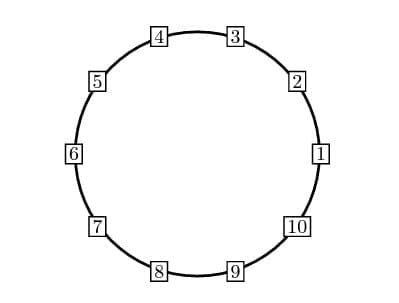This is a very beautiful sample problem from ISI MStat PSB 2013 Problem 10. It's based mainly on counting and following the norms stated in the problem itself. Be careful while thinking !

## Problem- ISI MStat PSB 2013 Problem 10

There are 10 empty boxes numbered 1,2,......,10 placed sequentially on a circle as shown in the figure,

We perform 100 independent trials. At each trial one box is selected with probability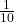and a ball is placed in each of the two neighboring boxes of the selected one.

Define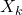be the number of balls in thebox at the end of 100 trials.

(a) Find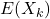for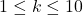.

(b) Find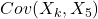for.

### Prerequisites

Counting principles

Binomial Distribution

Independence of Events

## Solution :

At first this problem may seem a bit of complex, but when one get to see the pattern it starts unfolding. For his types of problem, I find a useful technique is to follow the picture, in this case the picture is being provided, (if not draw it yourself !!)

Given,: # balls in thebox at the end of 100 trials.

So, the possible values ofare 0,1,2,.....,100. and the probability that at the jth trial a ball will added to the kth box is, (why??) .

Now,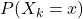=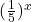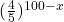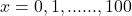Clearly,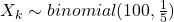, from here one can easily find out the the expectation of. But have you thought like this ??

Now, notice that after every slelection of box, 2 balls are added in the system, so at the end of the 10th trial, there will be 200 balls distributed in the system.

So,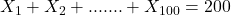, which implies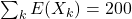, due to symmetry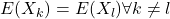, So,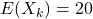.

(b) Now this part is the cream of this problem, first notice that number of balls in kth box, is dependent on the number of balls in the (k-2)th and (k+2)th box, and vice versa, So,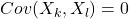if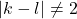.

So,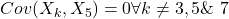, so we just need to find theand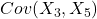, and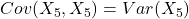.

Now it is sufficient to find the covariance of any of the the above mentioned covariances, as both are symmetric and identical to each other. But for the finding say, lets look whats happening in each trial more closely,

let,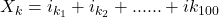where ,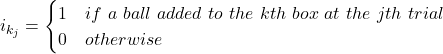So, clearly,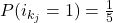; j=1,2,....,100.

So,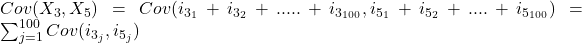, [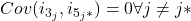, why ?? ].

So,.

similarly,also, and its easy to find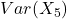, so I leave it as an exercise. So,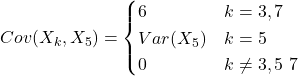. Hence we are done !!

## Food For Thought

Wait, lets imagine, these boxes are interchanged in such a way that the hth box is replaced with the kth (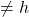) box, this has been done for all possible pairs of (h,k), Now can you show that all there are precisely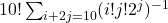number of arrangements possible ?

Now imagine, in a game there are 1 balls each in every box( boxes are arranged identically as shown in the question), you pick up the ball from the first box and put it into the 2nd one, now you can't take out any ball from a box in which you just put a ball, so you pick a ball from the 3rd box and put it into the 4th and you go on like this, taking a ball frim the ith box and put it into the (i+1)th box, but cant empty the box you just filled. Now, again after the first round you remove the empty boxes and do the same thing again and again, till all the balls are not accumulated in a single box. Which box you think will contain all the balls after you run this process finitely many time ?? If you are in this lottery and you are to choose a bix before this game begin, which box you must choose ??

if the coordinator of the game, starts with any ith box how should your strategy change ?? Give it a thought !!

For help, look for Josephus Problem, you may be moved by it's beauty !

## Subscribe to Cheenta at Youtube

This is a very beautiful sample problem from ISI MStat PSB 2013 Problem 10. It's based mainly on counting and following the norms stated in the problem itself. Be careful while thinking !

## Problem- ISI MStat PSB 2013 Problem 10

There are 10 empty boxes numbered 1,2,......,10 placed sequentially on a circle as shown in the figure,

We perform 100 independent trials. At each trial one box is selected with probabilityand a ball is placed in each of the two neighboring boxes of the selected one.

Definebe the number of balls in thebox at the end of 100 trials.

(a) Findfor.

(b) Findfor.

### Prerequisites

Counting principles

Binomial Distribution

Independence of Events

## Solution :

At first this problem may seem a bit of complex, but when one get to see the pattern it starts unfolding. For his types of problem, I find a useful technique is to follow the picture, in this case the picture is being provided, (if not draw it yourself !!)

Given,: # balls in thebox at the end of 100 trials.

So, the possible values ofare 0,1,2,.....,100. and the probability that at the jth trial a ball will added to the kth box is, (why??) .

Now,=Clearly,, from here one can easily find out the the expectation of. But have you thought like this ??

Now, notice that after every slelection of box, 2 balls are added in the system, so at the end of the 10th trial, there will be 200 balls distributed in the system.

So,, which implies, due to symmetry, So,.

(b) Now this part is the cream of this problem, first notice that number of balls in kth box, is dependent on the number of balls in the (k-2)th and (k+2)th box, and vice versa, So,if.

So,, so we just need to find theand, and.

Now it is sufficient to find the covariance of any of the the above mentioned covariances, as both are symmetric and identical to each other. But for the finding say, lets look whats happening in each trial more closely,

let,where ,So, clearly,; j=1,2,....,100.

So,, [, why ?? ].

So,.

similarly,also, and its easy to find, so I leave it as an exercise. So,. Hence we are done !!

## Food For Thought

Wait, lets imagine, these boxes are interchanged in such a way that the hth box is replaced with the kth () box, this has been done for all possible pairs of (h,k), Now can you show that all there are preciselynumber of arrangements possible ?

Now imagine, in a game there are 1 balls each in every box( boxes are arranged identically as shown in the question), you pick up the ball from the first box and put it into the 2nd one, now you can't take out any ball from a box in which you just put a ball, so you pick a ball from the 3rd box and put it into the 4th and you go on like this, taking a ball frim the ith box and put it into the (i+1)th box, but cant empty the box you just filled. Now, again after the first round you remove the empty boxes and do the same thing again and again, till all the balls are not accumulated in a single box. Which box you think will contain all the balls after you run this process finitely many time ?? If you are in this lottery and you are to choose a bix before this game begin, which box you must choose ??

if the coordinator of the game, starts with any ith box how should your strategy change ?? Give it a thought !!

For help, look for Josephus Problem, you may be moved by it's beauty !

## Subscribe to Cheenta at Youtube

This site uses Akismet to reduce spam. Learn how your comment data is processed.

### Knowledge Partner